## Background and purposes

Partition tables of MaxCompute  refer to the partition spaces specified during table creation. This refers certain fields in the table as partition columns.  When using data, if you mention the name of the partition you want to access, you can only read data from the corresponding partition. This eliminates need to scan the whole table, helping improve processing efficiency and reduce costs.

Partition pruning is to specify the filtering conditions for partition columns so that only part of partition data  in the table is read during SQL execution. This prevents data errors and resource waste caused by full table scan. Partition pruning seems simple, but actually partition failure often occurs. This article uses examples to introduce how to solve common problems.

## Problem examples

The following figure shows the partitions of the test table test_part_cut.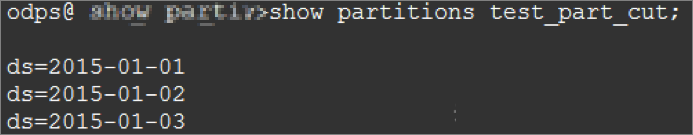Run the following SQL code:

``````
select count(*)
from test_part_cut
where ds= bi_week_dim('20150102');

--bi_week_dim is a user defined function. The return format is (year,sequential number of the week).
--If the date is normal, the system checks whether the date belongs to weeks of the input year when taking Thursday as the start day of a week. For example, if 20140101 is input, 2013,52 is returned because January 1, 2014 is Wednesday and considered as the last week of 2013.  If 20150101 is input ,  2015,1 is returned.
--If 20151231 is input, because December 31, 2015 is Thursday and is in the same week as January 1, 2016 ,  2016,1 is returned.``````

The returned result of bi_week_dim(‘20150102’) is 2015,1 and does not conform to the partition values of the test_part_cut table. Generally, we think that the preceding SQL statements  do not read any partition, but, actually, the SQL statements read all partitions in the test_part_cut table. The following figure shows the LogView: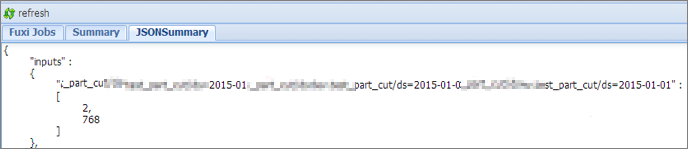In the preceding figure, the SQL statements read all partitions in the test_part_cut table when executed.

The preceding example shows that though easy-to-use, partition pruning often fails.  Therefore, this article focuses on the following aspects:

• Verify whether partition pruning in SQL statements takes effect.

• Know common scenarios that cause failed partition pruning.

## Verify whether partition pruning takes effect

You can run the explain command and view SQL execution plans to check whether partition pruning in the SQL statements takes effect.

• Effect of failed partition pruning

``````
explain
select seller_id
from xxxxx_trd_slr_ord_1d
where ds=rand();``````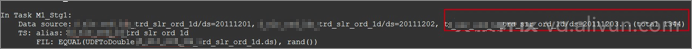The red box in the preceding figure shows that all the 1,344 partitions of the xxxxx_trd_slr_ord_1d table are read.

• Effect of successful partition pruning

``````
explain
select seller_id
from xxxxx_trd_slr_ord_1d
where ds='20150801';``````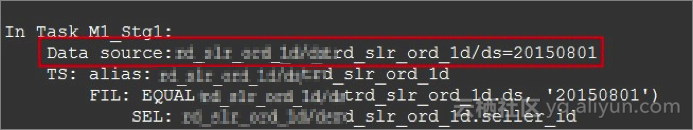The red box in the preceding figure shows that only the 20150801 partition of the xxxxx_trd_slr_ord_1d table is read.

## Analysis on scenarios of failed partition pruning

Partition pruning sometimes fails when custom functions or part of system functions are used,  and may also fail in the Where clause when the Join clause for joining is used.  These two scenarios are explained in the following examples.

Failed partition pruning caused by custom functions

If partition pruning conditions contain custom functions, partition pruning fails. However, some system functions may also cause partition pruning to fail. Therefore, considering the limit on partition values, you must run the  explain command to view the SQL statement execution plans and verify that partition pruning has taken effect when unusual functions are used.

``````
explain
select ...
from xxxxx_base2_brd_ind_cw
where ds = concat(SPLIT_PART(bi_week_dim(' \${bdp.system.bizdate}'), ',', 1), SPLIT_PART(bi_week_dim(' \${bdp.system.bizdate}'), ',', 2))``````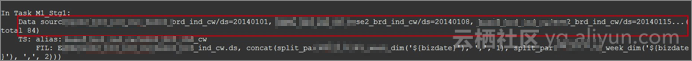In the preceding SQL statements, the custom functions used in partition pruning trigger full table scan.

Failed partition pruning caused by the Join clause

In SQL statements,  when you use the Join clause for joining, if partition pruning conditions are placed in the on clause, the partition pruning takes effect. If the conditions are placed in the Where clause, the partition pruning takes effect for the primary table, and does not apply to the foreign table.  The three Join clauses  are described as follows.

• Left Outer Join

• Place all partition pruning conditions in the on clause

``````
explain
select a.seller_id
,a.pay_ord_pbt_1d_001
from xxxxx_trd_slr_ord_1d a
left outer join
xxxxx_seller b
on a.seller_id=b.user_id
and a.ds='20150801'
and b.ds='20150801';``````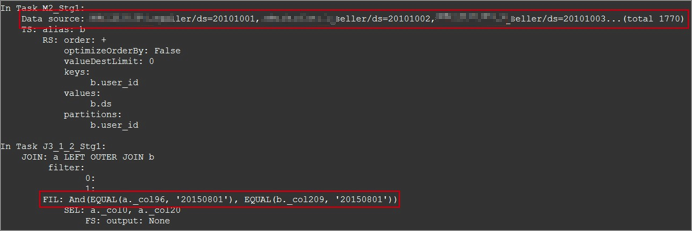As shown in the preceding figure, the left table is under full table scan, and only partition pruning for the right table takes effect.

• Place partition pruning conditions in the where clause

``````
explain
select a.seller_id
,a.pay_ord_pbt_1d_001
from xxxxx_trd_slr_ord_1d a
left outer join
xxxxx_seller b
on a.seller_id=b.user_id
where a.ds='20150801'
and b.ds='20150801';``````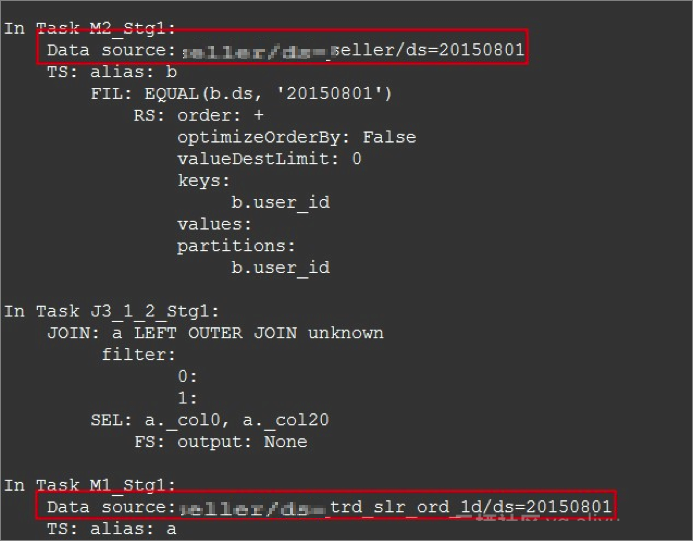As shown in the preceding figure, partition pruning for both the two tables takes effect.

• Right Outer Join

Similar to Left Outer Join, if partition pruning conditions are in the on clause, the conditions take effect only for the left table of Right Outer Join. If conditions are in the where clause,  the conditions take effect for both the two tables.

• Full Outer Join

Partition pruning conditions take effect only in the where clause, and fail in the on clause.

## Impact and consideration

• Partition pruning failure has serious results, which can be hardly discovered by users.  Therefore, we recommend that you check for failed partition pruning when submitting the code.

• More efforts are needed to solve the problem that custom functions cannot be used for partition pruning.